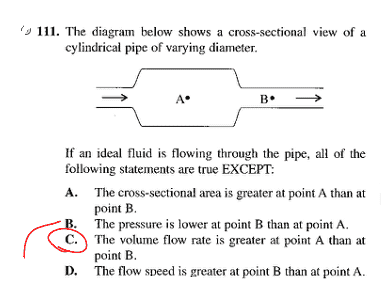# Ideal fluids, bernouilli's law

lovelyrwwrI do not understand why B is not true. There is much greater fluid molecules above point A, and given P = density x g x depth, pressure should be greater at point A.....making B true. But then again if u use poiseuilles' law for nonideal fluids you see that in order to keep flow rate Q constant, if radius is larger, then pressure must be smaller at point A....making B untrue. Could you pls explain how to recincile these cinflictinf cinclusions? Thank u so much !

Last edited:

Legaldose
If you wanna get mathematical, pressure can be defined as force per unit area, so

$$P = \frac{F}{A}$$

In the case of your picture, the cross sectional area of A is larger than B, so that would mean the pressure is less at A than at B

•1 person
lovelyrwwr
Thank u for your reply :) but how come we can assume that force is equal at points A @nd B?

imiuru
If you wanna get mathematical, pressure can be defined as force per unit area, so

$$P = \frac{F}{A}$$

In the case of your picture, the cross sectional area of A is larger than B, so that would mean the pressure is less at A than at B

The explanation is misleading. One should not analyze the situation from this perspective because doing so will cause you to hold F constant, which doesn't make sense.

imiuru
View attachment 63024

There is much greater fluid molecules above point A, and given P = density x g x depth, pressure should be greater at point A.....making B true.

You are using an equation from fluid statics to analyze a dynamic situation.

In dynamic fluid, pressure is never simply density x g x depth. You can study bernoulli's equation and realize that there are static pressure, dynamic pressure and pressure caused by gravity.

Legaldose
Okay I guess it's a little more complicated than that, let's see:

You have your continuity equation for fluids

$$\rho_{1}A_{1}v_{1}=\rho_{2}A_{2}v_{2}$$

The problem says that this is an ideal fluid, so the fluid isn't compressible, so

$$\rho_{1}=\rho_{2}$$

and

$$A_{1}v_{1}=A_{2}v_{2}$$

And since A = F/P

$$\frac{F_{1}}{P_{1}}v_{1}=\frac{F_{2}}{P_{2}}v_{2}$$

From a simple thought experiment we can conclude that the velocities are different, think about putting your thumb over a running water hose. And we have already seen that the pressures are the same. This leaves us with

$$F_{1}v_{1}=F_{2}v_{2}$$

Since the velocities are different, we can conclude that in fact, the forces must be different if both sides of the equation are to be equal.

imiuru
$$\frac{F_{1}}{P_{1}}v_{1}=\frac{F_{2}}{P_{2}}v_{2}$$

From a simple thought experiment we can conclude that the velocities are different, think about putting your thumb over a running water hose. And we have already seen that the pressures are the same. This leaves us with

$$F_{1}v_{1}=F_{2}v_{2}$$

##P_{1} = P_{2}## ? How is it possible?

Legaldose
Oh wow I just realized I wrote that, allow me to slowly walk away, as I clearly don't know fluid mechanics :sly: I'll go ahead and blame the time of night

lovelyrwwr
Thank you both the disvussion was very helpful!!!!